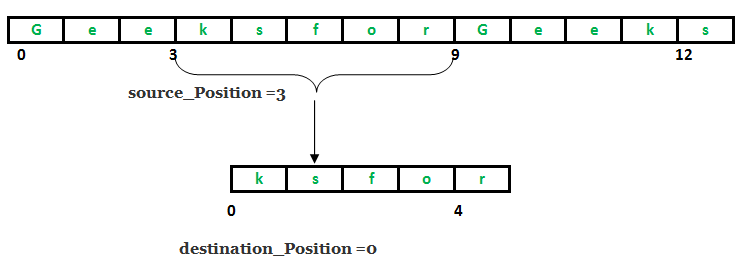Related Articles
System.arraycopy() in Java
• Difficulty Level : Easy
• Last Updated : 08 Aug, 2018

java.lang.System class provides useful methods for standard input and output, for loading files and libraries or to access externally defined properties. The java.lang.System.arraycopy() method copies a source array from a specific beginning position to the destination array from the mentioned position. No. of arguments to be copied are decided by len argument.
The components at source_Position to source_Position + length – 1 are copied to destination array from destination_Position to destination_Position + length – 1

Class Declaration

```public final class System
extends Object```Syntax :

```public static void arraycopy(Object source_arr, int sourcePos,
Object dest_arr, int destPos, int len)
Parameters :
source_arr : array to be copied from
sourcePos : starting position in source array from where to copy
dest_arr : array to be copied in
destPos : starting position in destination array, where to copy in
len : total no. of components to be copied.
```

Implementation

 `// Java program explaining System class method - arraycopy() ` `import` `java.lang.*; ` `public` `class` `NewClass ` `{ ` `    ``public` `static` `void` `main(String[] args) ` `    ``{ ` `        ``int` `s[] = { ``10``, ``20``, ``30``, ``40``, ``50``, ``60``, ``70``, ``80``, ``90``, ``100``}; ` `        ``int` `d[] = { ``15``, ``25``, ``35``, ``45``, ``55``, ``65``, ``75``, ``85``, ``95``, ``105``}; ` ` `  `        ``int` `source_arr[], sourcePos, dest_arr[], destPos, len; ` `        ``source_arr = s; ` `        ``sourcePos = ``3``; ` `        ``dest_arr = d; ` `        ``destPos = ``5``; ` `        ``len = ``4``; ` ` `  `        ``// Print elements of source ` `        ``System.out.print(``"source_array : "``); ` `        ``for` `(``int` `i = ``0``; i < s.length; i++) ` `            ``System.out.print(s[i] + ``" "``); ` `        ``System.out.println(``""``); ` ` `  `        ``System.out.println(``"sourcePos : "` `+ sourcePos); ` `        `  `        ``// Print elements of source ` `        ``System.out.print(``"dest_array : "``); ` `        ``for` `(``int` `i = ``0``; i < d.length; i++) ` `            ``System.out.print(d[i] + ``" "``); ` `        ``System.out.println(``""``); ` `        `  `        ``System.out.println(``"destPos : "` `+ destPos); ` `        `  `        ``System.out.println(``"len : "` `+ len); ` `        `  `        ``// Use of arraycopy() method ` `        ``System.arraycopy(source_arr, sourcePos, dest_arr,  ` `                                            ``destPos, len); ` `        `  `        ``// Print elements of destination after ` `        ``System.out.print(``"final dest_array : "``); ` `        ``for` `(``int` `i = ``0``; i < d.length; i++) ` `            ``System.out.print(d[i] + ``" "``); ` `    ``} ` `}  `

Output:

```source_array : 10 20 30 40 50 60 70 80 90 100
sourcePos : 3
dest_array : 15 25 35 45 55 65 75 85 95 105
destPos : 5
len : 4
final dest_array : 15 25 35 45 55 40 50 60 70 105
```

This article is contributed by Mohit Gupta. If you like GeeksforGeeks and would like to contribute, you can also write an article using contribute.geeksforgeeks.org or mail your article to contribute@geeksforgeeks.org. See your article appearing on the GeeksforGeeks main page and help other Geeks.